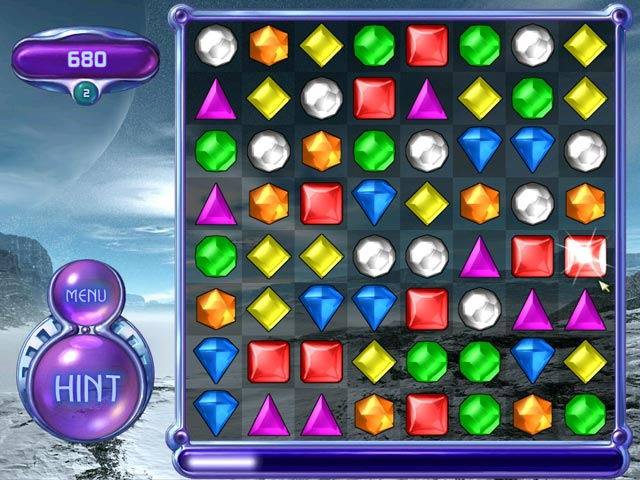# JS1K X part 1: Fruit Crush Saga

So, sadly 2019’s JS1K edition was the last one, so I decided to participate with two entries! This post is about Fruit Crush Saga.

## The game

I’m sure most people know Candy Crush Saga, a very popular cellphone game. It’s gameplay it’s quite close to Bejeweled of back in the day. Since nobody had made a version of that kind of game for the JS1K contest, I decided to take the challenge!Each of those jewel images easily weights qmore than 1Kb, so what to use? To me, the obvious choice was emojis, and the fruit emoji range is perfect for the task; I decided to use 🍓, 🥝, 🍉, 🍊, 🍇, 🥥 and 🍌, as they are quite visually distinct in most browsers.The rules are the same as Candy Crush Saga/Bejeweled; exchange two adjacent fruits vertically or horizontally, and if after the change there’s a group of adjacent fruits with 3 or more in a row or column, it’s removed, and new fruits will fall from the top. Chain reactions are really satisfying!

## Tricks

Nothing too exotic here.

• Instead of using the provided canvas, the code will build and inject HTML; I borrowed the concept from the excellent Tiny Chess entry by Óscar Toledo G.

• The board data is defined as a single array instead of a bidimensional one, which makes the code clearer but would make the code a bit bigger.

• For the highscore, `localStorage` is used in order to persist it; however, instead of the usual `getItem`/`setItem` API, it uses it as an object, which I wasn’t really aware was possible until I checked other JS1K entries. Also, the highscore key is just `9`, so no quotes are needed.

• Functions store their results in globals instead of returning a value.

## Commented source code

``````// Initializing variables
q=r=h=d=z=0,s=99,F=[];

// Find explodable fruits in current board, and store the positions in `o`
O=i=>{
o=[];
for(i=0;i<64;i++)i%8<6&&F[i]==F[i+1]&&F[i]==F[i+2]&&o.push(i,i+1,i+2),i<48&&F[i]==F[i+8]&&F[i]==F[i+16]&&o.push(i,i+8,i+16)
};

// Check if there's any available move; `e` will be `1` if the game should end
E=i=>{
e=1;
for(i=0;i<64;i++)j=F[i],i%8<7&&(F[i]=F[i+1],F[i+1]=j,O(),F[i+1]=F[i],F[i]=j,o.length&&(e=0)),F[i]=F[i+8],F[i+8]=j,O(),F[i+8]=F[i],F[i]=j,o.length&&(e=0)
};

// Explode fruits due to correct swap
X=i=>{
x=[];
for(i=0;i<64;i++)!F[63-i]&&(F[63-i]=i<56?(j=F[55-i],F[55-i]="",j):["🍓","🥝","🍉","🍊","🍇","🥥","🍌"][7*Math.random()|0],x.push(63-i));
};

// Animation that blinks the exploding fruits
Y=i=>{setTimeout(h&&(--h||(r+=5*o.length,o.map(i=>F[i]="")))||d&&d--?Y:X,25),B()};

// Show animation for wrong move, and restore state when finished playing
Q=i=>{setTimeout(q&&q--?Q:(F[l]=F[m],F[m]=k,X),99),B()};

// Interchange cell fruits
I=i=>{
m=S,l=s,s=99,
k=F[m],F[m]=F[l],F[l]=k,
O(),
B(),
o.length?setTimeout(X,25):(q=11,setTimeout(Q,99))
};

// Initialize board, ensuring there's at least one valid move
for(i=0;i<64||(E(),O(),o.length+e)&&!(i=0);i++)F[i]=["🍓","🥝","🍉","🍊","🍇","🥥","🍌"][7*Math.random()|0];

// Onscreen HTML board and cell rendering
B=i=>{
// Render every cell in the game
for(i=0;i<64;j+="<th width=64 height=64 onclick='s="+i+";i=S%8-s%8;j=S-s;(j*j-1||i*i-1)&&j*j-64?B():I()'bgcolor=#"+(i-s?1&(i+i/8|0)?443:554:990)+"><span style='position:relative;font-size:30px;top:-"+(d&&x.includes(i)&&d*8)+"px'>"+(4&h&&o.includes(i)?"":2&q&&(i==l||i==m)?"🙅":F[i])+(++i%8?"":"<tr>"))
S=s;

// Update highscore if needed
r<=localStorage||(z=localStorage=r);

// Render current score and highscore
b.innerHTML=j+"<th colspan=4><big>"+r+"<th colspan=4><big>🏆"+localStorage
};

// Start the game
B();
``````

## That’s all

This was a fun little game and something I had in my mind for a while, so I’m glad I was able to finally go and implement it for the last edition of JS1K. I’m always amazed on all that can fit in a single kilobyte of JavaScript!